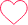# Java program to determine can all numbers of an array be made equal

## Check if all the numbers of array can be made equal in Java

Here, in this page we will discuss the program to check if all the numbers of array can be made equal in Java programming language. We are given with an integer array and need to print “Yes” or “No”.## Key Point

A positive integer number can be factorized as prime factors and written as 2a * 3b * 5c * 7d *…
We can multiply given numbers by 2 and 3 so we can increase a and b for them. So we can make all a and b equal by increasing them to the same big value.But we can’t change powers of other prime numbers so they must be equal from the beginning. We can check it by diving all numbers from input by two and by three as many times as possible. Then all of them must be equal.

## Algorithm

• Start iterating over the array.
• Run a loop till arr[i]%2=0, and inside that loop divide the arr[i] by 2.
• Run a loop till arr[i]%3=0, and inside that loop divide the arr[i] by 3.
• Check if arr[i] != arr, then return 0.
• Otherwise, return 1.

### Code in Java

Run
```//Write a program to check can all number of an array be made equal in Java

import java.util.*;
public class Main
{
public static boolean make_equal(int arr[], int n)
{
for (int i = 0; i < n; i++)
{
// Divide number by 2
while (arr[i] % 2 == 0)
arr[i] /= 2;

// Divide number by 3
while (arr[i] % 3 == 0)
arr[i] /= 3;
}
// Remaining numbers
for (int i = 1; i < n; i++)
if (arr[i] != arr){
return false;
}
return true;
}

public static void main (String[] args)
{
int arr[] = { 50, 100, 75 };

int m = arr.length;

if (make_equal(arr, m))
System.out.print("Yes");
else
System.out.print("No");
}

}```

`Yes`

### Related Banners

Get PrepInsta Prime & get Access to all 200+ courses offered by PrepInsta in One Subscription

### 3 comments on “Java program to determine can all numbers of an array be made equal”

•Rudransh

def equal(a,l):
for i in range(l):
while a[i]%2==0:
a[i]/=2
while a[i]%3==0:
a[i]/=3
if a[i]!=a:
return False
return True
a=list(map(int,input().split()))
l=len(a)
if equal(a,l):
print(“yes”)
else:
print(“no”)0
•Ayushi

#Python Program

size = int(input(“Enter array size: “))
arr = []
for i in range(size):
arr.append(int(input()))

for i in range(size):
while arr[i]%2 == 0:
arr[i] /= 2

while arr[i]%3 == 0:
arr[i] /= 3

for i in range(size):
if arr[i] != arr:
print(“N0”)
break
else:
print(“Yes”)1
•DHANANJAY

———-CODE IN PYTHON————-
def equal(n):
for i in range(len(n)):
while(n[i]%2==0):
n[i] /= 2
while(n[i]%3==0):
n[i] /= 3
for i in range(len(n)):
if(n[i]!=n):
return False
return True
n = list(map(int,input().split()))
if(equal(n)):
print(“Yes”)
else:
print(“No”)0